Question

# The temperature of a chemical reaction oscillates regularly and symmetrically. The lowest temperature is 50°C at...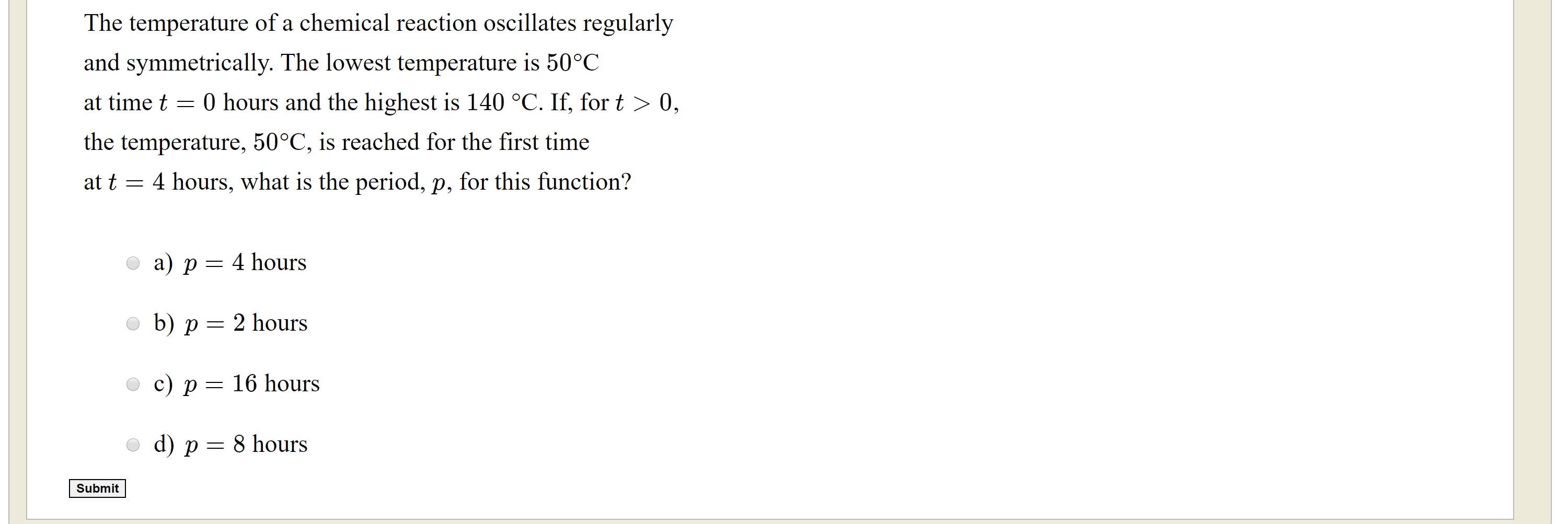The temperature of a chemical reaction oscillates regularly and symmetrically. The lowest temperature is 50°C at time t = 0 hours and the highest is 140 °C. If, for t > 0, the temperature, 50°C, is reached for the first time at t = 4 hours, what is the period, p, for this function? o a) p = 4 hours o b) p = 2 hours O c) p = 16 hours od) p = 8 hours Submit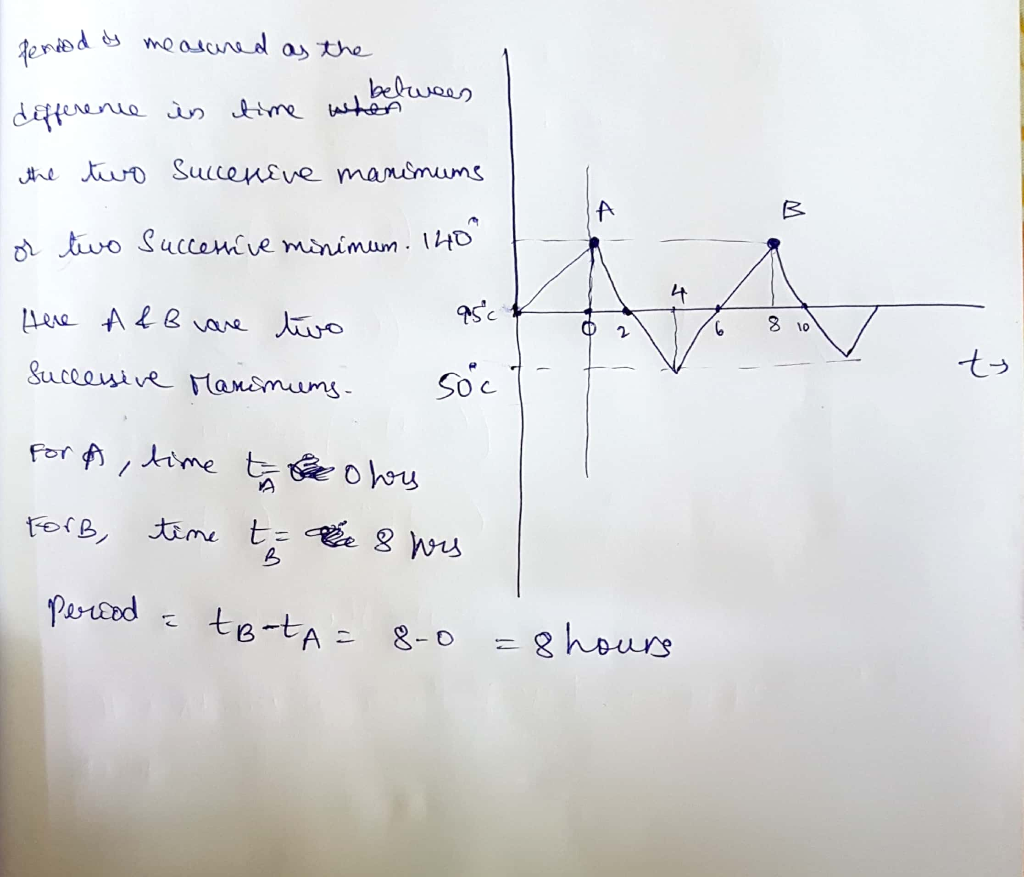#### Earn Coins

Coins can be redeemed for fabulous gifts.

Similar Homework Help Questions
• ### 3. (4 marks) A desert temperature oscillates between-5°C at 4am to 35°C at 4pm, then down again to-5°C at 4am the next...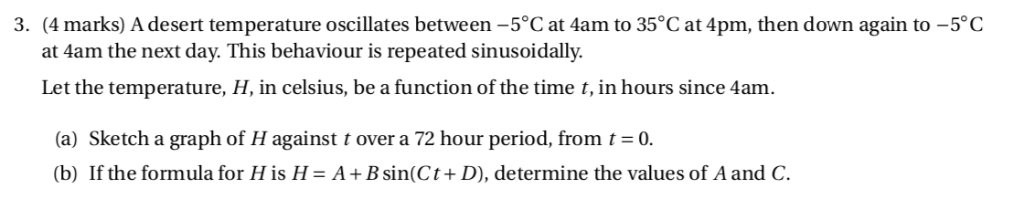3. (4 marks) A desert temperature oscillates between-5°C at 4am to 35°C at 4pm, then down again to-5°C at 4am the next day. This behaviour is repeated sinusoidally. Let the temperature, H, in celsius, be a function of the time t,in hours since 4am. (a) Sketch a graph of H against t over a 72 hour period, from t0 (b) If the formula for H is H- A + B sin(Ct + D), determine the values of A and C....

• ### Du Find a possible formula for the trigonometric function whose values are in the following table....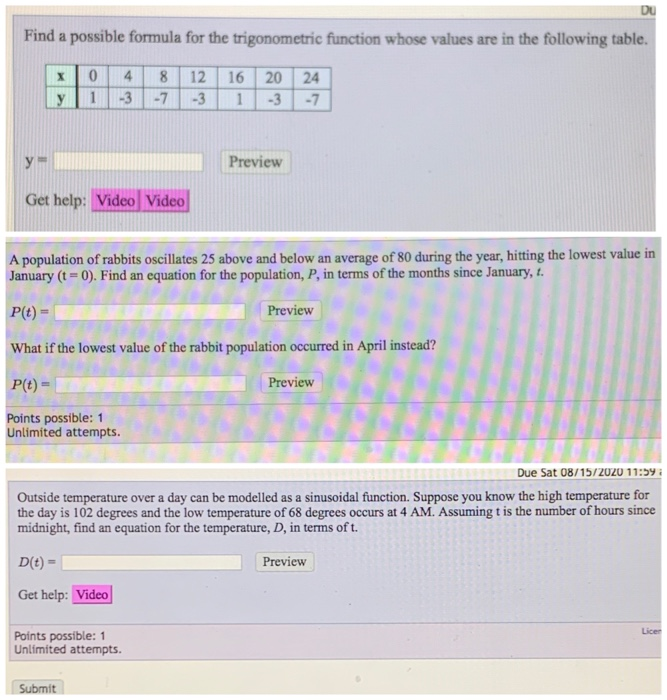Du Find a possible formula for the trigonometric function whose values are in the following table. 4. 0 1 8 - 7 12 16 20 24 -3 -7 у -3 -3 1 Preview Get help: Video Video A population of rabbits oscillates 25 above and below an average of 80 during the year, hitting the lowest value in January (t = 0). Find an equation for the population, P, in terms of the months since January, t. P(t) - Preview...

• ### A chemical engineer is studying the following reaction: H2(0)+C(9)- 2HCl(9) t the temperature the engineer picks,...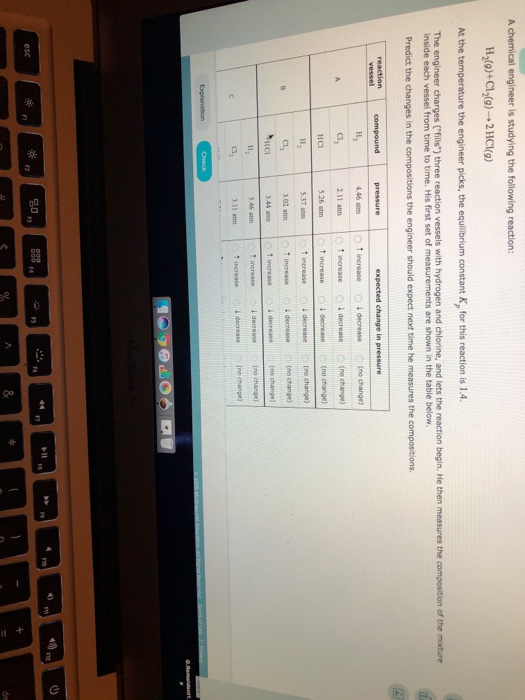A chemical engineer is studying the following reaction: H2(0)+C(9)- 2HCl(9) t the temperature the engineer picks, the equilibrium constant K, for this reaction is 1.4. The engineer charges (ills") three reaction vessels with hydrogen and chlorine, and lets the reaction begin. He then measures the composition of the mixture inside each vessel from time to time. His first set of measurements are shown in the table below. Predict the changes in the compositions the engineer should expect next time he...

• ### 1. Outside temperature over a day can be modeled as a sinusoidal function. Suppose you know...

1. Outside temperature over a day can be modeled as a sinusoidal function. Suppose you know the high temperature for the day is 82 degrees and the low temperature of 68 degrees occurs at 3 AM. Assuming t is the number of hours since midnight, find an equation for the temperature, D, in terms of t. D(t)= 2. A population of rabbits oscillates 18 above and below an average of 62 during the year, hitting the lowest value in January...

• ### The Temperature Dependence of Chemical Equilibrium For an equilibrium reaction: A + B C + D...The Temperature Dependence of Chemical Equilibrium For an equilibrium reaction: A + B C + D Given the above relationship, use the graph to identify which of the following statements are "true" or "false" in this specific case. All statements refer to the forward reaction (proceeding left to right, as given) corresponding to the equilibrium constant K. 1)The reaction is spontaneous at the highest temperatures but not at lower temperatures. 2)deltaS° for the reaction is negative. 3)deltaG° is negative at...

• ### Suppose the reaction temperature X (in °C) in a certain chemical process has a uniform distribution...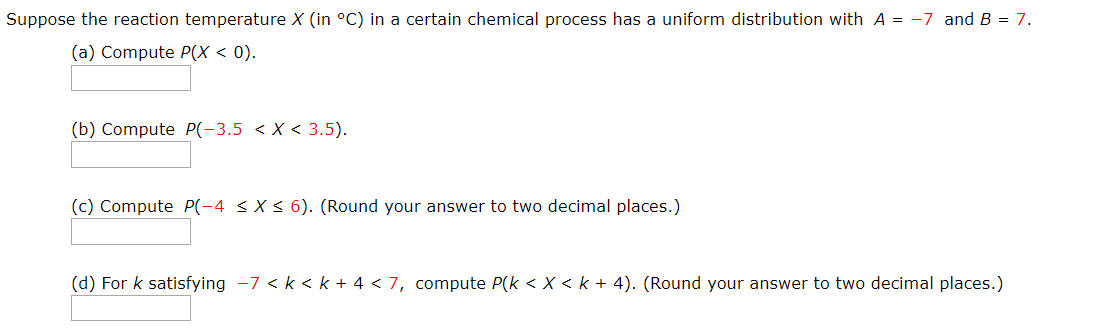Suppose the reaction temperature X (in °C) in a certain chemical process has a uniform distribution with A = -7 and B = 7. (a) Compute P(x < 0). (b) Compute P(-3.5 < X < 3.5). (c) Compute P(-4 SX36). (Round your answer to two decimal places.) (d) For k satisfying -7<k<k+ 4 < 7, compute P(k <x<k + 4). (Round your answer to two decimal places.)

• ### Reaction Rates and Temperature 31 Review Constants i Peric The rate constant of a chemical reaction...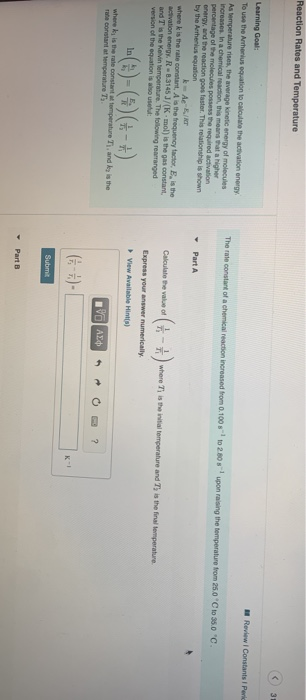Reaction Rates and Temperature 31 Review Constants i Peric The rate constant of a chemical reaction increased from 0.100 - 2005 - pon raising the temperature from 250 C 350 C Learning Goal To use the Athenius equation to calculate the activation energy As temperature rises, the average kinetic energy of molecules increases. In a chemical reaction, this means at a higher percentage of the molecules possess the required activation energy and the reaction goes faster. This relationship is shown...

• ### A chemical engineer is studying the following reaction: H (9)+1 (9) 2 Hl(0) At the temperature...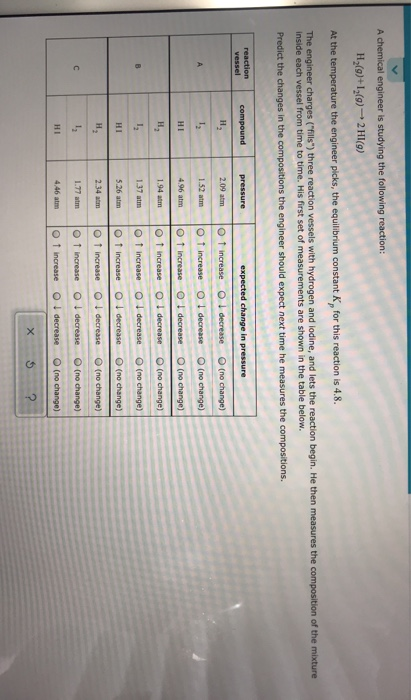A chemical engineer is studying the following reaction: H (9)+1 (9) 2 Hl(0) At the temperature the engineer picks, the equilibrium constant for this reaction is 4.8. The engineer charges ("fills") three reaction vessels with hydrogen and lodine, and lets the reaction begin. He then measures the composition of the mixture Inside each vessel from time to time. His first set of measurements are shown in the table below. Predict the changes in the compositions the engineer should expect next...

• ### Submit Question 33 of 50 For the chemical reaction H2 (g)+ F2 (g) 2 HF (g)...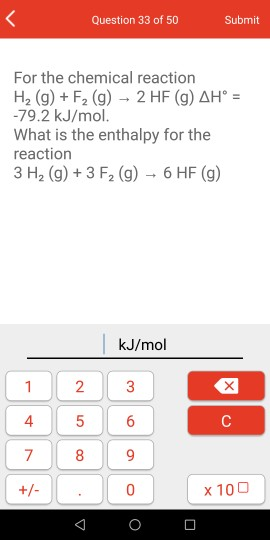Submit Question 33 of 50 For the chemical reaction H2 (g)+ F2 (g) 2 HF (g) AH = -79.2 kJ/mol. What is the enthalpy for the reaction 3 H2 (g)+3 F2 (g) 6 HF (g) kJ/mol 1 2 3 4 5 6 C 8 +- 0 x 100 O 7

• ### Required information A chemical reaction is run 12 times, and the temperature x, (in °C) and...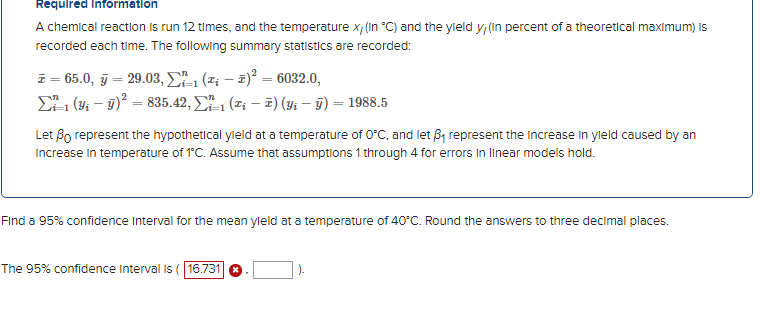Required information A chemical reaction is run 12 times, and the temperature x, (in °C) and the yield y (in percent of a theoretical maximum) is recorded each time. The following summary statistics are recorded: 7=65.0, y = 29.03, 1-1 (; - 2)2 = 6032.0, 2-1 (y; – 5)2 = 835.42, 1-1 (; – 7) (yi - y) = 1988.5 Let Bo represent the hypothetical yield at a temperature of O°C, and let By represent the increase in yleld caused...